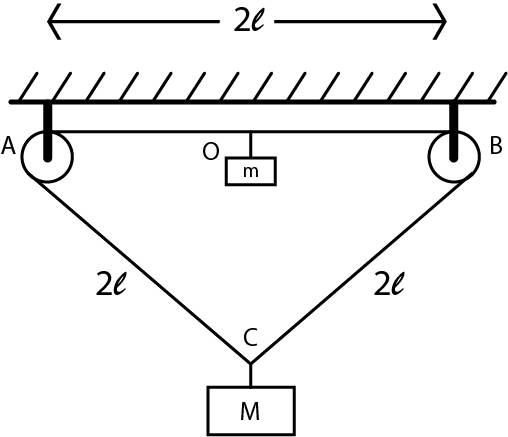# Give me ID Card-2!A triangular loop $ABC$ of light string is passed over two small frictionless pulleys A and B $(AB = BC = AC = 2l)$

Two masses $m$ and $M$ are attached at the midpoint $O$ of $AB$ and at point $C$, respectively. If $m$ is released, $m$ & $M$ cross each other at any point $P$ as shown in the figure. Find the minimum value of $\dfrac {m}{M}$ so that they cross each other.

×